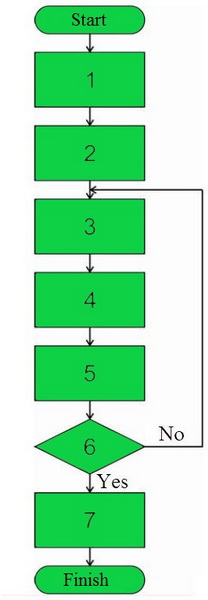Counсil of young scientists BSUIR
Совет молодых ученых БГУИР
RU | EN

# Method of rejecting the integrated schemes after effects of static electricity by the contact dischargePrepared by: Gennadiy Piskun, Ph.D., Associate Professor, Associate Professor of Information Systems Design Department.

The object of the invention is to develop a method for rejecting integrated circuits after the action of static electricity by the method of a contact discharge based on the construction of an imitation model of the article under investigation in which the system of heat conduction and electrical conductivity equations is calculated, which makes it possible to predict the failure of an IC at different voltages of an acting contact discharge.
The proposed method is carried out as follows (Figure):

1. Definition of areas (current-conducting elements of the IC), in which the temperature distribution will be calculated, as well as the development of a model of a certain geometric shape (length, width).
2. Statement and formalization of problems on the description of the processes of heat and electrical conductivity in conductive elements of the IC.
3. Specify the numerical values of the parameters for each area of the IC.
4. Setting the boundary conditions for each area of the IC.
5. Calculation and modeling of temperature distribution in current-carrying elements of integrated circuits in the software complex.
6. Analysis of calculation results.
No: in case the obtained calculation results, performed in clause 5, do not satisfy the conditions of the developer, then the transition to item 3 is carried out.
Yes: the obtained calculation results, performed in clause 5, satisfy the conditions of the developer.
7. Development of measures to optimize the parameters of conductive elements of the IC.

A distinctive feature of the claimed method of rejecting integrated circuits after the action of static electricity by the contact discharge method is the construction in a software package of a model in which the temperature distribution in conducting conductors of IC and semiconductor devices is calculated and modeled.